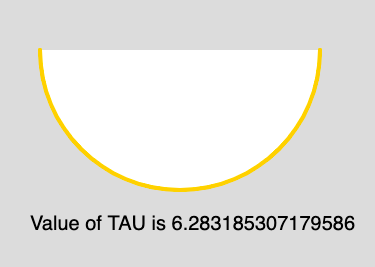Related Articles

# p5.js | Constants | TAU

• Last Updated : 12 Apr, 2019

The TAU is a mathematical constant and it is the alias of TWO_PI. The value of TAU is 6.28318530717958647693. It is twice the ratio of the circumference of a circle to its diameter.

Syntax:

`TAU`

Below program illustrates the usage of TAU in p5.js:

Example: This example illustrates the TAU constant.

 `function` `setup() {`` ` `    ``// Create Canvas of size 880*300``    ``createCanvas(880, 300);``}`` ` `function` `draw() {``     ` `    ``//Set the background Color``    ``background(220);``     ` `    ``//Set the stroke color``    ``stroke(255, 204, 0);``     ` `    ``//Set the stroke weight``    ``strokeWeight(4);``     ` `    ``// Use of constant TAU``    ``arc(180, 50, 280, 280, 0, TAU/2); ``     ` `    ``noStroke();``     ` `    ``// Set the font size``    ``textSize(20);``     ` `    ``// Display result``    ``text(``"Value of TAU is "``+ TAU, 30, 230);``}`

Output:Reference: https://p5js.org/reference/#/p5/TAU

My Personal Notes arrow_drop_up